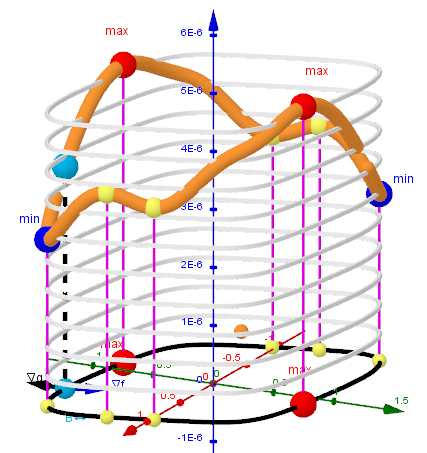# Lagrange Multipliers ● Example 7

Extremizing f(x, y)=exp(-x² - 2y²) (x² + 3y²) with the constraint condition: g(x,y):=x⁴ + 2y⁶ + x y - 0.8 = 0 . CAS and ggB solutions are compared. The critical points of the corresponding Lagrangian, its global maximum and minimum are determined.Curve of Intersection of two surfaces: f (x, y) and g(x,y).